The OpenFOAM Foundation
SphericalTensor< Cmpt > Class Template Reference

Templated 3D SphericalTensor derived from VectorSpace adding construction from 1 component, element access using th ii() member function and the inner-product (dot-product) and outer-product operators. More...

Inheritance diagram for SphericalTensor< Cmpt >: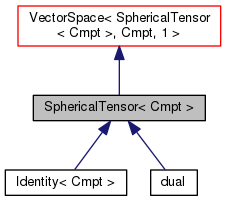[legend]
Collaboration diagram for SphericalTensor< Cmpt >: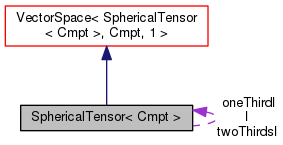[legend]

## Public Types

enum  components { II }
Component labeling enumeration. More...

typedef SphericalTensor< labellabelType
Equivalent type of labels used for valid component indexing. More...Public Types inherited from VectorSpace< SphericalTensor< Cmpt >, Cmpt, 1 >
typedef VectorSpace< SphericalTensor< Cmpt >, Cmpt, Ncmpts > vsType
VectorSpace type. More...

typedef Cmpt cmptType
Component type. More...

## Public Member Functions

SphericalTensor ()
Construct null. More...

SphericalTensor (const Foam::zero)
Construct initialized to zero. More...

template<class Cmpt2 >
SphericalTensor (const VectorSpace< SphericalTensor< Cmpt2 >, Cmpt2, 1 > &)
Construct given VectorSpace. More...

SphericalTensor (const Cmpt &tii)
Construct given the component. More...

SphericalTensor (Istream &)
Construct from Istream. More...

const Cmpt & ii () const

Cmpt & ii ()

const SphericalTensor< Cmpt > & T () const
Transpose. More...Public Member Functions inherited from VectorSpace< SphericalTensor< Cmpt >, Cmpt, 1 >
VectorSpace ()
Construct null. More...

VectorSpace (const Foam::zero)
Construct initialized to zero. More...

VectorSpace (Istream &)
Construct from Istream. More...

VectorSpace (const VectorSpace< SphericalTensor< Cmpt >, Cmpt, Ncmpts > &)
Construct as copy. More...

VectorSpace (const VectorSpace< Form2, Cmpt2, Ncmpts > &)
Construct as copy of a VectorSpace with the same size. More...

const Cmpt & component (const direction) const

Cmpt & component (const direction)

void component (Cmpt &, const direction) const

void replace (const direction, const Cmpt &)

const ConstBlock< SubVector, BStart > block () const

const Cmpt & operator[] (const direction) const

Cmpt & operator[] (const direction)

void operator= (const VectorSpace< SphericalTensor< Cmpt >, Cmpt, Ncmpts > &)

void operator= (const Foam::zero)

void operator+= (const VectorSpace< SphericalTensor< Cmpt >, Cmpt, Ncmpts > &)

void operator-= (const VectorSpace< SphericalTensor< Cmpt >, Cmpt, Ncmpts > &)

void operator*= (const scalar)

void operator/= (const scalar)

## Static Public Attributes

static const direction rank = 2
Rank of SphericalTensor is 2. More...

static const SphericalTensor I

static const SphericalTensor oneThirdI

static const SphericalTensor twoThirdsIStatic Public Attributes inherited from VectorSpace< SphericalTensor< Cmpt >, Cmpt, 1 >
static const direction dim
Dimensionality of space. More...

static const direction nComponents
Number of components in this vector space. More...

static const direction mRows

static const direction nCols

static const char *const typeName

static const char *const componentNames []

static const SphericalTensor< Cmpt > zero

static const SphericalTensor< Cmpt > one

static const SphericalTensor< Cmpt > max

static const SphericalTensor< Cmpt > min

static const SphericalTensor< Cmpt > rootMax

static const SphericalTensor< Cmpt > rootMinStatic Public Member Functions inherited from VectorSpace< SphericalTensor< Cmpt >, Cmpt, 1 >
static direction size ()
Return the number of elements in the VectorSpace = Ncmpts. More...

static SphericalTensor< Cmpt > uniform (const Cmpt &s)
Return a VectorSpace with all elements = s. More...Public Attributes inherited from VectorSpace< SphericalTensor< Cmpt >, Cmpt, 1 >
Cmpt v_ [Ncmpts]
The components of this vector space. More...

## Detailed Description

### template<class Cmpt> class Foam::SphericalTensor< Cmpt >

Templated 3D SphericalTensor derived from VectorSpace adding construction from 1 component, element access using th ii() member function and the inner-product (dot-product) and outer-product operators.

Source files

Definition at line 51 of file SphericalTensor.H.

## Member Typedef Documentation

 typedef SphericalTensor

Equivalent type of labels used for valid component indexing.

Definition at line 59 of file SphericalTensor.H.

## Member Enumeration Documentation

 enum components

Component labeling enumeration.

Enumerator
II

Definition at line 76 of file SphericalTensor.H.

## Constructor & Destructor Documentation

 SphericalTensor ( )
inline

Construct null.

Definition at line 31 of file SphericalTensorI.H.

Referenced by SphericalTensor< Cmpt >::SphericalTensor().

Here is the caller graph for this function: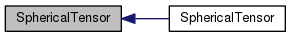SphericalTensor ( const Foam::zero )
inline

Construct initialized to zero.

Definition at line 36 of file SphericalTensorI.H.

References SphericalTensor< Cmpt >::SphericalTensor().

Here is the call graph for this function: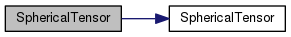SphericalTensor ( const VectorSpace< SphericalTensor< Cmpt2 >, Cmpt2, 1 > & vs )
inline

Construct given VectorSpace.

Definition at line 45 of file SphericalTensorI.H.

 SphericalTensor ( const Cmpt & tii )
inline

Construct given the component.

Definition at line 54 of file SphericalTensorI.H.

 SphericalTensor ( Istream & is )
inline

Construct from Istream.

Definition at line 61 of file SphericalTensorI.H.

## Member Function Documentation

 const Cmpt & ii ( ) const
inline

Definition at line 70 of file SphericalTensorI.H.

Here is the caller graph for this function: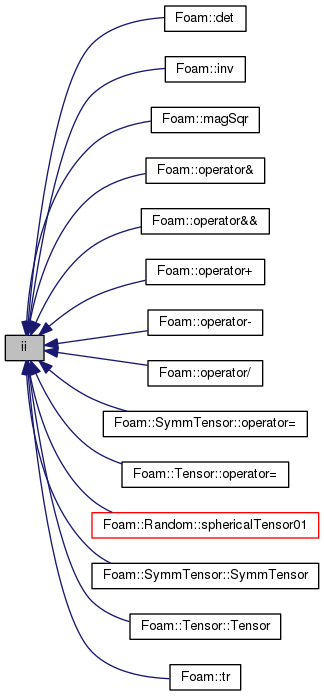Cmpt & ii ( )
inline

Definition at line 77 of file SphericalTensorI.H.

 const Foam::SphericalTensor< Cmpt > & T ( ) const
inline

Transpose.

Definition at line 85 of file SphericalTensorI.H.

## Member Data Documentation

 const direction rank = 2
static

Rank of SphericalTensor is 2.

Definition at line 65 of file SphericalTensor.H.

 const Foam::sphericalTensor I
static

Definition at line 70 of file SphericalTensor.H.

 const Foam::sphericalTensor oneThirdI
static

Definition at line 71 of file SphericalTensor.H.

 const Foam::sphericalTensor twoThirdsI
static

Definition at line 72 of file SphericalTensor.H.

The documentation for this class was generated from the following files: